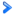Hi fellow wiki editors!

To help newly registered users get more familiar with the wiki (and maybe older users too) there is now a {{Welcome to the wiki}} template. Have a look at it and feel free to add it to new users discussion pages (and perhaps your own).

I have tried to keep the template short, but meaningful. /Johan G

# Numbers in Nasal

As one would expect, there are numbers in Nasal.

## Representation types

### Decimal

The most basic number system is the decimal system, based on the number 10. Decimal numbers can be written as integers, floating point numbers, or in exponential form.

#### Integer

Integers can be written as below:

```var number = 1234; # 1,234
number = -1234; # -1,234```

#### Floating point

Floating point numbers can be written as below:

```var number = 1.234;
number = -1.234;```

#### Exponential

Number written in exponential form (powers of ten) take the form of `number[E/e]power`. The number part may be either an integer or floating point number, and either negative or positive. The indicate that the number is in exponential form, either capital or lowercase `e` may be used. The power part must be an integer, and may be either positive or negative.

```var number = 12e6; # 12,000,000
number = 4E-2; # 0.04
number = 6.674E-11; # 0.00000000006674 (gravitational constant)```

Numbers may also be written as hexadecimal numbers (0 to 9 and A to F). The digits must be preceded by a zero and lowercase "x" (`0x`). Any letters in the digits may be either capital or lowercase. Nasal does not support floating point numbers in hexadecimal form, so this representation is limited to integers.

```var number = 0x2f; # 47
number = 0x2F; # 47
number = -0x2f; # -47```

### Octal

 Note  Support for octal numbers was introduced in FlightGear v3.2.

The final type is octal numbers (0 to 7). The digits must be preceded by a zero and lowercase "o" (`0o`). This does not support floating point numbers as well.

```var number = 0o23; # 19
number = -0o23; # -19```

## OperatorsSee Nasal Operators for the main article about this subject.

All the usual operators work as expected. All the number representation types may be combined in expressions, for example:

```# 5e-2 = 0.05, 0x2f = 47, 0o37 = 31
print(2.35 + 5e-2 - 0x2f * 0o37 / -2); # prints 730.9```

## Related content

• `printf()` and `sprintf()` functions